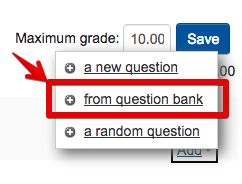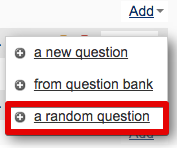Once a Quiz has been created and you are at the stage of adding questions, it is simple to randomly select questions from a question bank. All questions that exist in a unit from other Quiz or Lesson activities will be available to select, whether you knowingly added them to the question bank or not.

### Adding a question from a question bank3.
4. Select the category from the dropdown.

5. Tick the question(s) you would like to add to the quiz.

6. Click the 'Add selected questions to the quiz' button.

Random questions allows different students to receive different quiz questions. It is important to categorise questions if you want to create a quiz with random questions.

You might not, for example, put questions of a different type in the same category. Otherwise, one student might get a random 'essay' type question whereas a different student might end up with a 'true/false' type question.

It is also important to have more questions in a category than the number of random questions (in that category) in the quiz. For example, you might have a category 'Knowledge questions' that contains 20 questions. In the quiz you might select five questions from this category. Each student will randomly be allocated five of the 20 'Knowledge questions'.3.
4. Select the category of question and then the number of questions from that category you would like in the quiz.

5. Click the 'Add random question' button.